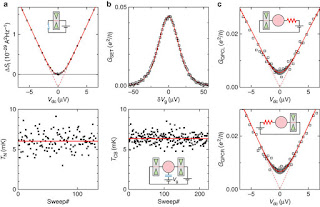## Friday, October 21, 2016

### Measuring temperature at the milliKelvin scale

How do we tell the temperature of some piece of material?  I've written about temperature and thermometry a couple of times before (here, here, here).  For ordinary, every-day thermometry, we measure some physical property of a material or system where we have previously mapped out its response as a function of temperature.  For example, near room temperature liquid mercury expands slightly with increasing $T$.  Confined in a thin glass tube, the length of a mercury column varies approximately linearly with changes in temperature, $\delta \ell \sim \delta T$.  To do primary thermometry, we don't want to have some empirical calibration - rather, we want to measure some physical property for which we think we have a complete understanding of the underlying physics, so that $T$ can be inferred directly from the measured quantity and our theoretical expressions, with no adjustable parameters.  This is particularly important at very low temperatures, thousandths of a Kelvin above absolute zero, where the number of things that we can measure is comparatively limited, and tiny flows of power (from our measurements, say) can actually produce large percentage temperature changes.

This recent paper shows a nice example of applying three different primary thermometry techniques to a single system, a puddle of electrons confined in 2d at a semiconductor interface, at about 6 mK.  This is all the more impressive because of how easy it is to inadvertently heat up electrons in such 2d layers.  All three techniques rely on our understanding of how electrons behave at low temperatures.  According to our theory of electrons in metals (which these 2d electrons are, as far as physicists are concerned), as a function of energy, electrons are spread out in a characteristic way, the Fermi-Dirac distribution.  From the theory side, we know this functional form exactly (figure from that wikipedia link).  At low temperatures, all of the electronic states below a highest-filled-state are full, and all above are empty.  As $T$ is increased, the electrons smear out into higher energy states, as shown.  The three effects measured in the experiment all depend on $T$ through this electronic distribution:Fig. 2 from the paper, showing excellent, consistent agreement between experiment and theory, showing electron temperatures  of ~ 6 mK.
• Current noise in a quantum point contact, the fluctuations in the average current.  For this particular device, where conduction takes place through a small, controllable number of quantum channels, we think we understand the situation completely.  There is a closed-form expression for what the noise should do as a function of average current, with temperature as the only adjustable parameter (once the conduction has been measured).
• "Coulomb blockade" in a quantum dot.  Conduction through a puddle of electrons connected to input and output electrodes by tunneling barriers ("pinched off" versions of the point contacts) shows a very particular form of current-voltage characteristic that is tunable by a nearby gate electrode.   The physics here is that, because of the mutual repulsion of electrons, it takes energy (supplied by either a voltage source or temperature) to get charge to flow through the puddle.  Again, once the conduction has been measured, there is a closed-form expression for what the conductance should do as a function of that gate voltage.
• "Environmental" Coulomb blockade in a quantum dot.  This is like the situation above, but with one of the tunnel barriers replaced by a controlled resistor.  Again, there is an expression for the particular shape of the $I-V$ curve where the adjustable parameter is $T$.
As shown in the figure (Fig. 2 from the paper - open access and also available on the arxiv), the theoretical expressions do a great job of fitting the data, and give very consistent electron temperatures down to 0.006 K.  It's a very impressive piece of work, and I encourage you to read it - look at Fig. 4 if you're interested in how challenging it is to cool electrons in these kinds of devices down to this level.